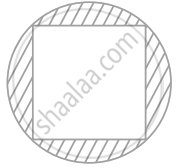# In figure, a square of diagonal 8 cm is inscribed in a circle. Find the area of the shaded region. - Mathematics

Sum
Theorem

In figure, a square of diagonal 8 cm is inscribed in a circle. Find the area of the shaded region.#### Solution

Let us take a be the side of square.

Diameter of a circle = Diagonal of the square = 8 cm

In right angled triangle ABC,

Using Pythagoras theorem,

(AC)^2 = (AB)^2 + (BC)^2

(8)^2 = a^2 + a^2

64 = 2a^2

a^2 = 32

Area of square = a2

= 32  cm2

Radius of the circle = "Diameter"/2

Area of the circle = pir^2

= pi(4)^2

= 16 cm2

So, the area of the shaded region = Area of circle – Area of square

The area of the shaded region = 16pi - 32

= 16 xx (22/7) - 32

= 128/7

= 18.286 cm2

Concept: Area of Circle
Is there an error in this question or solution?

#### APPEARS IN

NCERT Mathematics Exemplar Class 10
Chapter 11 Area Related To Circles
Exercise 11.3 | Q 2 | Page 125

Share